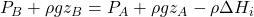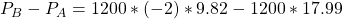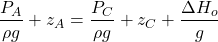## 注册化工工程师执业考试2018真题 14-15

• Post author:
• Post category:深冷技术

### 题目14

A 2900 B 2356 C 2312 D2030

### 解答Q = 20m3/h
H = 28-0.01 * 20 * 20 =24

Q = 20 * 0.7 = 14
H = 8 + 0.04 * 14 * 14 = 15.84N2 = 2355.97

### 题目15

90°弯头 0.75
Y型过滤器 0.88A 28.55 54 B 24.72 51 C 28.55 -44 D 24.72 -47

### 解答= (0.04 * 5 /0.08 + 0.17+0.88+1.0 * 2+ 0.75 ) * 2.39 * 2.39 / 2
= 17.99= -45156 Pa.G= (0.04 * 20 /0.08 +6.4 +2.0 + 0.75 * 5) * 2.39 * 2.39 / 2
= 63.26H= 15+1 + (63.26+17.99)/9.8
= 24.29